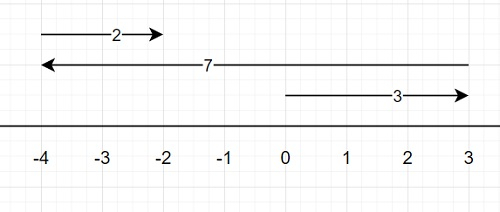# Program to count how many blocks are covered k times by walking in Python

Suppose we have two lists called walks and target. At the beginning we are at position 0 in a one-dimensional line. Now |walks[i]| represents the number of steps have been walked. And when walk[i] is positive then indicates walked right, and negative for left. When we walk, we move one block, that is the next or previous integer position. We have to find the number of blocks that's been walked on at least target number of times.

So, if the input is like walks = [3, -7, 2] target = 2, then the output will be 5, from the following figure, we can see [0, 1], [1, 2], [2, 3], [-4, -3], [-3, -2] are covered k = 2 times.To solve this, we will follow these steps −

• pos := 0
• jumps := a hash map, where default value is 0 when key is not present
• for each dist in walks, do
• jumps[pos] := jumps[pos] + 1 if dist > 0 otherwise -1
• jumps[pos + dist] := jumps[pos + dist] - 1 if dist > 0 otherwise -1
• pos := pos + dist
• lastpos := 0
• level := 0
• total := 0
• for each position pos, and value val in the sorted key-value pairs of jumps, do
• if level >= target, then
• total := total + pos - lastpos
• level := level + val
• lastpos := pos

## Example

Let us see the following implementation to get better understanding −

from collections import defaultdict
def solve(walks, target):
pos = 0
jumps = defaultdict(int)
for dist in walks:
jumps[pos] += 1 if dist > 0 else -1
jumps[pos + dist] -= 1 if dist > 0 else -1
pos += dist
lastpos = level = total = 0
for pos, val in sorted(jumps.items()):
if level >= target:
total += pos - lastpos
level += val
lastpos = pos

walks = [3, -7, 2]
target = 2
print(solve(walks, target))

## Input

[3, -7, 2], 2

## Output

5